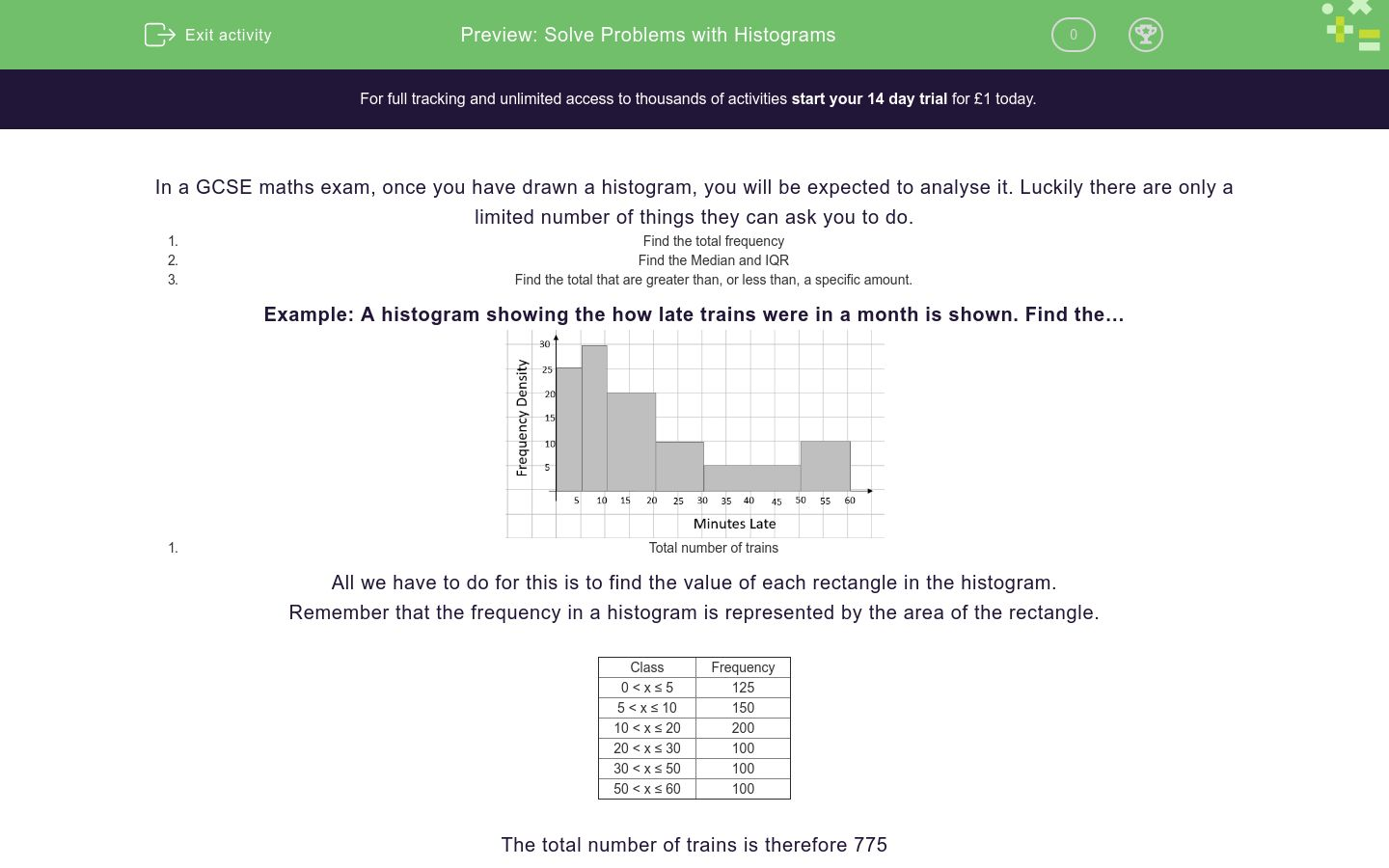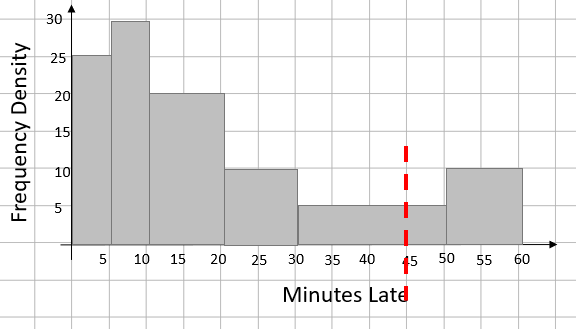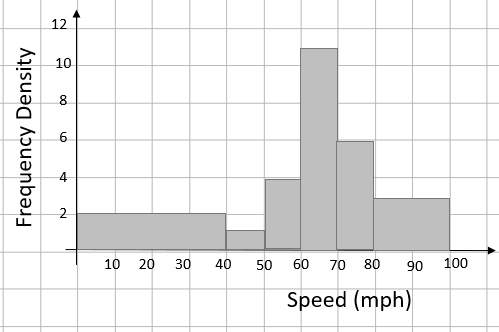# Solve Problems with Histograms

In this worksheet, students will practice reading data from histogramsKey stage:  KS 4

GCSE Subjects:   Maths

GCSE Boards:   AQA, Eduqas, Pearson Edexcel, OCR

Curriculum topic:   Statistics

Curriculum subtopic:   Statistics, Interpreting and Representing Data

Difficulty level:### QUESTION 1 of 10

In a GCSE maths exam, once you have drawn a histogram, you will be expected to analyse it. Luckily there are only a limited number of things they can ask you to do.

1. Find the total frequency
2. Find the Median and IQR
3. Find the total that are greater than, or less than, a specific amount.

Example: A histogram showing the how late trains were in a month is shown. Find the…1. Total number of trains

All we have to do for this is to find the value of each rectangle in the histogram.

Remember that the frequency in a histogram is represented by the area of the rectangle.

 Class Frequency 0 < x ≤ 5 125 5 < x ≤ 10 150 10 < x ≤ 20 200 20 < x ≤ 30 100 30 < x ≤ 50 100 50 < x ≤ 60 100

The total number of trains is therefore 775

1. Estimate the median and interquartile range (IQR) for how late the trains were.

We know there are 775 trains in the survey. This means the median would be at the 388th position, the lower quartile would be at 194th number and the upper quartile at 582nd position.

Finding an estimate of the Median (388th position).

We can count through the frequencies and find that the 388th position is in the group 10 < x ≤ 20. The question is how far into this group?

The first two bars use 125 + 150 = 275 of the positions so we need to go 113 (388 – 275) positions into this group.

As the group represents 200 trains, we need to go 113/200 into the group which is 10 wide.

If we calculate 113/200 x 10 we get 5.65

Adding this onto the start of the group gives 10 + 5.65

So the estimated median is 15.65 minutes.

If we repeat this process for the lower and upper quartiles, we get LQ = 7.3 and UQ of 31.4 minutes.

This gives an IQR of 31.4 – 7.3 = 24.1 minutes

1. Find the percentage of trains that were more than 45 minutes late.

If we draw a line showing the point we are looking at we get…As we’re looking for the percentage that are greater than 45 minutes, we need to find the frequency represented by the bars to the right of the line.

The first one is ¼ of the group 30 < x ≤ 50 which will be 25 and the second group is all of the group 50 < x ≤ 60 (100 trains)

With our total of 125 / 775, this is 16.13% (to 2 dp)

To find the frequency of a single group in a histogram, we _________ the class width by the frequency density.

The speeds of cars passing a bridge on a dual carriageway are recorded and put into a histogram.Find the total number of cars that were seen.

The speeds of cars passing a bridge on a dual carriageway are recorded and put into a histogram.How many cars were traveling between 30 and 80 mph?

60

240

300

e speeds of cars passing a bridge on a dual carriageway are recorded and put into a histogram.Find an estimate of the median speed of the cars. Give your answer to 1 dp. Do not put units in your answer.

All Year 11 students in a school took a mock maths paper and their scores turned into a histogram.Find the total number of pupils in year 11

All Year 11 students in a school took a mock maths paper and their scores turned into a histogram.Estimate the IQR of the pupils scores. Give all your answers to 1 dp

All Year 11 students in a school took a mock maths paper and their scores turned into a histogram.80% of the students passed. What was the pass mark?

People at a gym were surveyed about how far they traveled to get to the gym. If we know that 30 people traveled between 10 and 12 miles, how many were asked in total.Estimate the median and IQRValue Median LQ UQ IQR

How many people travelled less than 7.5 km to the gym.• Question 1

To find the frequency of a single group in a histogram, we _________ the class width by the frequency density.

multiply
times
EDDIE SAYS
The key difference for histograms is that the frequency of the group is represented by the area of the bar.
• Question 2

The speeds of cars passing a bridge on a dual carriageway are recorded and put into a histogram.Find the total number of cars that were seen.

EDDIE SAYS
Remember that we find the total number in the survey by finding the area of each bar and adding them together.
• Question 3

The speeds of cars passing a bridge on a dual carriageway are recorded and put into a histogram.How many cars were traveling between 30 and 80 mph?

240
EDDIE SAYS
We are looking for between 30 and 80 so we illustrate this on the diagram.All we need to do now is find the areas for each bar between the two dashed lines.
• Question 4

e speeds of cars passing a bridge on a dual carriageway are recorded and put into a histogram.Find an estimate of the median speed of the cars. Give your answer to 1 dp. Do not put units in your answer.

64.5
EDDIE SAYS
We have 360 cars in total so the median will be the 180th. Working through the frequencies, we see that is 50 into the groups 60 – 70 50/110 x 10 = 4.5 Adding this onto the total gives 65.4 mph
• Question 5

All Year 11 students in a school took a mock maths paper and their scores turned into a histogram.Find the total number of pupils in year 11

300
EDDIE SAYS
Remember that we find the total number in the survey by finding the area of each bar and adding them together.
• Question 6

All Year 11 students in a school took a mock maths paper and their scores turned into a histogram.Estimate the IQR of the pupils scores. Give all your answers to 1 dp

32.5
EDDIE SAYS
The first step we have to do is to find the position of the LQ and UQ As we have 300 pupils, the LQ will be the 75th position and the UQ will be the 225th position. The 75th position will be 15 into the group 80 – 90. As this bar is worth 40. We need to go 15/40 in. 15/40 x 10 = 3.75 Adding this onto the starting point of the bar gives a LQ of 83.75 Using this process again for the UQ gives 116.25 We can then simply find the IQR
• Question 7

All Year 11 students in a school took a mock maths paper and their scores turned into a histogram.80% of the students passed. What was the pass mark?

80
EDDIE SAYS
If 80% passed, this means that 20% failed. 20 % of the students is 60 students (coincidentally this is the value of the first bar) so the pass mark must have been…
• Question 8

People at a gym were surveyed about how far they traveled to get to the gym. If we know that 30 people traveled between 10 and 12 miles, how many were asked in total.230
EDDIE SAYS
The biggest problem we have here is that we don’t have the frequency density. We know that frequency density = frequency / class width If we put in the info we have for between 10 and 12 km. We get a frequency density of 15. From that, we can work out the frequency density scale.Once we have this, we can just work out the area of each bar and add them together.
• Question 9

Estimate the median and IQRValue Median LQ UQ IQR
EDDIE SAYS
Let’s look at the process for finding the LQ. We have 230 people, this means the LQ is the 57.5th value. Counting through the frequencies gives that the LQ is 27.5 into the group 6 – 7 The group 6-7 is worth 40 people so we need to go 27.5 out of 40 into this. 27.5/40 x 1 = 0.6875 Adding this onto the start of the group gives 6.7 (rounded). We can do something similar for both the median and UQ
• Question 10

How many people travelled less than 7.5 km to the gym.100
EDDIE SAYS
Less than 7.5 km means all the area to the left of the dashed line.The first two bars are worth 20 and 50. The area in the third box that is to the left of the line is 0.5 x 40 = 20 people.
---- OR ----

Sign up for a £1 trial so you can track and measure your child's progress on this activity.

### What is EdPlace?

We're your National Curriculum aligned online education content provider helping each child succeed in English, maths and science from year 1 to GCSE. With an EdPlace account you’ll be able to track and measure progress, helping each child achieve their best. We build confidence and attainment by personalising each child’s learning at a level that suits them.

Get started